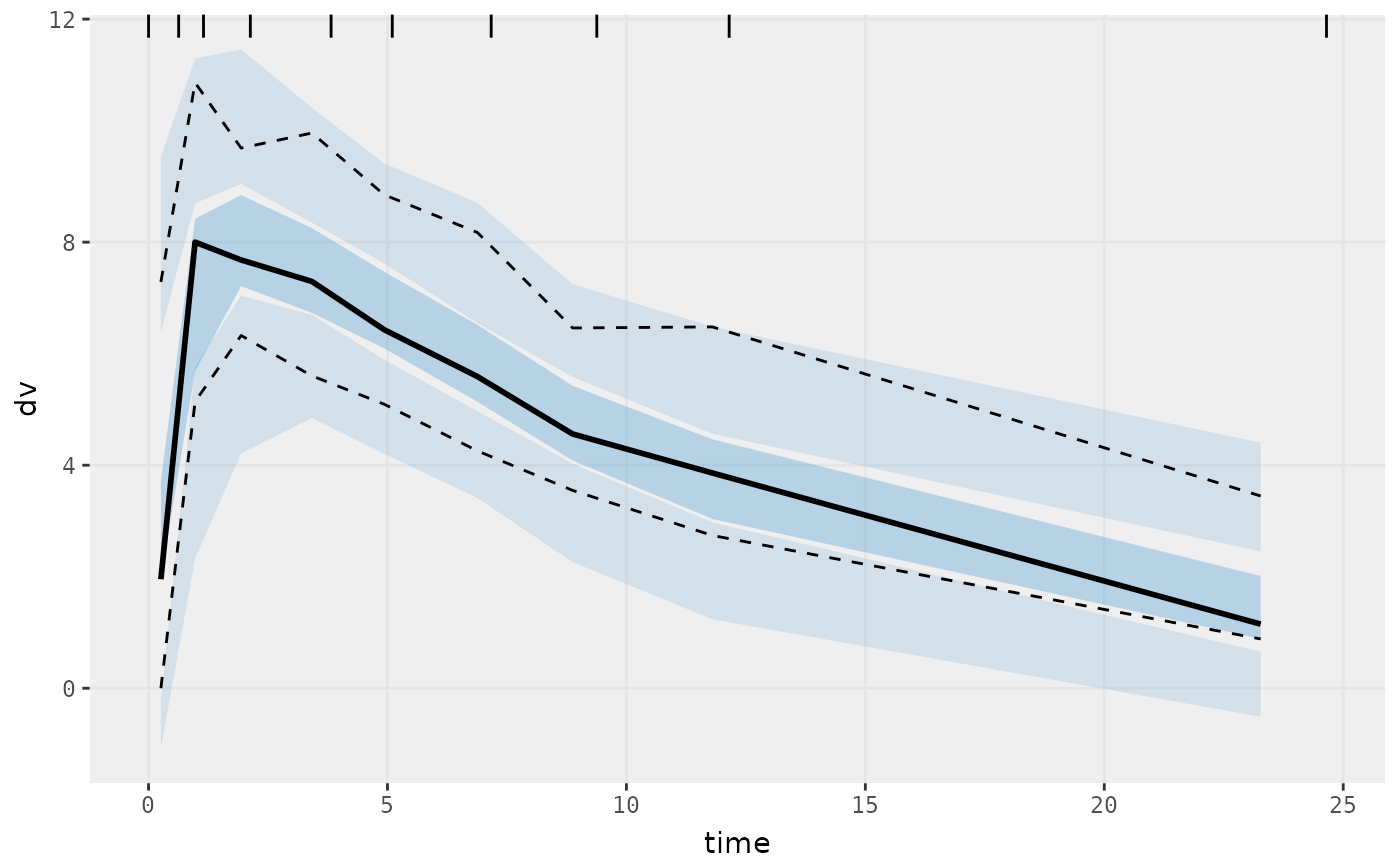VPC based on ui model

## Usage

vpcPlot(
fit,
data = NULL,
n = 300,
bins = "jenks",
n_bins = "auto",
bin_mid = "mean",
show = NULL,
stratify = NULL,
pred_corr = FALSE,
pred_corr_lower_bnd = 0,
pi = c(0.05, 0.95),
ci = c(0.05, 0.95),
uloq = NULL,
lloq = NULL,
log_y = FALSE,
log_y_min = 0.001,
xlab = NULL,
ylab = NULL,
title = NULL,
smooth = TRUE,
vpc_theme = NULL,
facet = "wrap",
scales = "fixed",
labeller = NULL,
vpcdb = FALSE,
verbose = FALSE,
...,
seed = 1009
)

## Arguments

fit

nlmixr2 fit object

data

this is the data to use to augment the VPC fit. By default is the fitted data, (can be retrieved by getData), but it can be changed by specifying this argument.

n

Number of VPC simulations. By default 100

bins

either "density", "time", or "data", "none", or one of the approaches available in classInterval() such as "jenks" (default) or "pretty", or a numeric vector specifying the bin separators.

n_bins

when using the "auto" binning method, what number of bins to aim for

bin_mid

either "mean" for the mean of all timepoints (default) or "middle" to use the average of the bin boundaries.

show

what to show in VPC (obs_dv, obs_ci, pi, pi_as_area, pi_ci, obs_median, sim_median, sim_median_ci)

stratify

character vector of stratification variables. Only 1 or 2 stratification variables can be supplied.

pred_corr

perform prediction-correction?

pred_corr_lower_bnd

lower bound for the prediction-correction

pi

simulated prediction interval to plot. Default is c(0.05, 0.95),

ci

confidence interval to plot. Default is (0.05, 0.95)

uloq

Number or NULL indicating upper limit of quantification. Default is NULL.

lloq

Number or NULL indicating lower limit of quantification. Default is NULL.

log_y

Boolean indicting whether y-axis should be shown as logarithmic. Default is FALSE.

log_y_min

minimal value when using log_y argument. Default is 1e-3.

xlab

label for x axis

ylab

label for y axis

title

title

smooth

"smooth" the VPC (connect bin midpoints) or show bins as rectangular boxes. Default is TRUE.

vpc_theme

theme to be used in VPC. Expects list of class vpc_theme created with function vpc_theme()

facet

either "wrap", "columns", or "rows"

scales

either "fixed" (default), "free_y", "free_x" or "free"

labeller

ggplot2 labeller function to be passed to underlying ggplot object

vpcdb

Boolean whether to return the underlying vpcdb rather than the plot

verbose

show debugging information (TRUE or FALSE)

...

Additional arguments passed to nlmixr2plot::vpcPlot().

seed

an object specifying if and how the random number generator should be initialized

## Value

Simulated dataset (invisibly)

## Author

Matthew L. Fidler

## Examples

# \donttest{
one.cmt <- function() {
ini({
## You may label each parameter with a comment
tka <- 0.45 # Log Ka
tcl <- log(c(0, 2.7, 100)) # Log Cl
## This works with interactive models
## You may also label the preceding line with label("label text")
tv <- 3.45; label("log V")
## the label("Label name") works with all models
eta.ka ~ 0.6
eta.cl ~ 0.3
eta.v ~ 0.1
})
model({
ka <- exp(tka + eta.ka)
cl <- exp(tcl + eta.cl)
v <- exp(tv + eta.v)
})
}

fit <- nlmixr2est::nlmixr(one.cmt, nlmixr2data::theo_sd, est="focei")
#>
#>
#>
#> ℹ parameter labels from comments will be replaced by 'label()'
#> → pruning branches (if/else) of full model...
#> ✔ done
#> → calculate jacobian
#> → calculate ∂(f)/∂(η)
#> → calculate ∂(R²)/∂(η)
#> → finding duplicate expressions in inner model...
#> → optimizing duplicate expressions in inner model...
#> → finding duplicate expressions in EBE model...
#> → optimizing duplicate expressions in EBE model...
#> → compiling inner model...
#>
#> ✔ done
#> → finding duplicate expressions in FD model...
#> → compiling EBE model...
#>
#> ✔ done
#> → compiling events FD model...
#>
#> ✔ done
#> → Calculating residuals/tables
#> ✔ done
#> → compress origData in nlmixr2 object, save 5952
#> → compress parHist in nlmixr2 object, save 2072
#> Warning: gradient problems with initial estimate and covariance; see \$scaleInfo
#> Warning: last objective function was not at minimum, possible problems in optimization
#> Warning: ETAs were reset to zero during optimization; (Can control by foceiControl(resetEtaP=.))
#> Warning: initial ETAs were nudged; (can control by foceiControl(etaNudge=., etaNudge2=))

vpcPlot(fit)
#># }